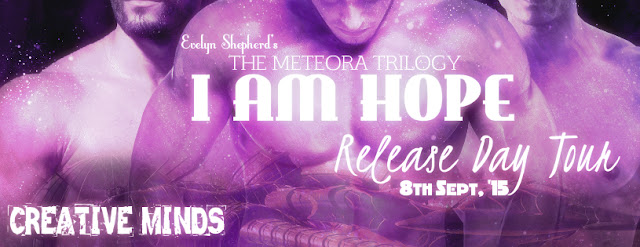## Tuesday, September 8, 2015

### I Am Hope by Evelyn Sheperd - Release Day Tour with Giveaway•=•=•=•=•=•=•=•=•=•=•=•=•=•=•=•=•=•=•=•=•=•=•=•=•=•=•=•=•=•
Evelyn Shepherd’s next book in her THE METEORA TRILOGY series titled I AM HOPE is OUT today.
•=•=•=•=•=•=•=•=•=•=•=•=•=•=•=•=•=•=•=•=•=•=•=•

Publisher: Loose Id
Cover Artist: Valerie Tibbs
Length: 70,000 words

Blurb:

The undead were only the beginning.

Topher, Jesse, and Sawyer thought their greatest threat were the Infected and Mutated that roam the world. As they struggle to reach the camp in Kill Devil Hills in North Carolina, they soon discover that the monstrous mutations of the undead are the least of their problems. Something else, something far more intelligent and lethal, is hunting them.

Topher knows that the only way humanity will survive is to find a cure for the strange infection that’s ravaged the world. He’s on the brink of discovering it; but the further his research goes, the more he realizes that curing the world of the infection may mean losing Sawyer.

The three will fight to stay together, and Topher will have to make the ultimate choice: cure the world or save his lover?

•=•=•=•=•=•=•=•=•=•=•=•=•=•=•=•=•=•=•=•=•=•=•=•
EXCERPT

I knuckled the sleep from my eyes and hurriedly dressed. Jesse was out the door by the time I pulled my shirt on. I followed him into the main compartment, Sawyer behind me. Rio sat on the couch next to Jaden, who was curled up with his blanket. On the table was a camping lamp, which filled the RV with yellow light.

“What’s going on?” I asked, straightening my shirt.

“Something is going down with Jane,” Rio said. “I heard it out in the truck.”

Nash pulled Chloe into his arms and drew her away from the window. Jesse walked over to the dinette table and looked outside, then let out a string of curses. I nudged him over and looked out myself.

“Sweet Jesus,” I whispered. The blood chilled in my veins, turning into ice fractals that splintered through my mind. I gripped the table as I watched the genocide going on outside.
People were being dragged from their tents and herded together like sheep for slaughter. It was hard to see how many people had been gathered. Clouds shrouded the moon, and the fires had smoldered out, leaving the camp a battlefield of shadows.

And you will burn in a lake of fire, unless you repent and purge the sinners from your life. We must show God that we are believers and his servants. It’s the only way to find the path to salvation.

•=•=•=•=•=•=•=•=•=•=•=•=•=•=•=•=•=•=•=•=•=•=•=•

Evelyn Shepherd lives in Columbus, Ohio with two fat cats. Her time is split between writing and running a book/writing blog. She’s the author of the Theo Bourne Series, the best-selling Last Canticle, and the award-winning Meteora Trilogy.

||  Blog & Website  ||  Facebook Page  ||  Twitter  ||  Tumblr  ||  Email  ||

•=•=•=•=•=•=•=•=•=•=•=•=•=•=•=•=•=•=•=•=•=•=•=•

RAFFLECOPTER GIVEAWAY

Winner’s Prize: E-copies of I AM HERE (Book 1) and I AM ALIVE (Book 2)